### 3. COSMOLOGICAL PARAMETERS

The standard model for describing the global evolution of the Universe is based on two equations that make some simple, and hopefully valid, assumptions. If the Universe is isotropic and homogenous on large scales, the Robertson-Walker Metric,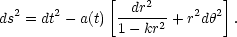(4)

gives the line element distance(s) between two objects with coordinates r,and time separation, t. The Universe is assumed to have a simple topology such that, if it has negative, zero, or positive curvature, k takes the value -1, 0, 1, respectively. These models of the Universe are said to be open, flat, or closed, respectively. The dynamic evolution of the Universe needs to be input into the Robertson-Walker Metric by the specification of the scale factor a(t), which gives the radius of curvature of the Universe over time - or more simply, provides the relative size of a piece of space at any time. This description of the dynamics of the Universe is derived from General Relativity, and is known as the Friedman equation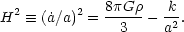(5)

The expansion rate of our Universe (H), is called the Hubble parameter (or the Hubble constant, H0, at the present epoch) and depends on the content of the Universe. Here we assume the Universe is composed of a set of components, each having a fraction,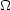i, of the critical density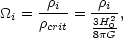(6)

with an equation of state which relates the density,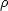i, and pressure, pi, as wi = pi /i. For example, wi takes the value 0 for normal matter, +1/3 for photons, and -1 for the cosmological constant. The equation of state parameter does not need to remain fixed; if scalar fields are present, the effective w will change over time. Most reasonable forms of matter or scalar fields have wi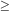- 1, although nothing seems manifestly forbidden. Combining Eqs. (4-6) yields solutions to the global evolution of the Universe .

The luminosity distance, DL, which is defined as the apparent brightness of an object as a function of its redshift z - the amount an object's light has been stretched by the expansion of the Universe - can be derived from Eqs. (4-6) by solving for the surface area as a function of z, and taking into account the effects of time dilation [25, 26, 50, 82] and energy dimunition as photons get stretched traveling through the expanding Universe. DL is given by the numerically integrable equation,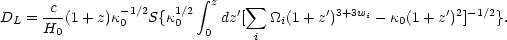(7)

S(x) = sin(x), x, or sinh(x) for closed, flat, and open models respectively, and the curvature parameter0, is defined as0 =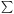ii - 1.

Historically, Eq. (7) has not been easily integrated and has been expanded in a Taylor series to give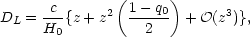(8)

where the deceleration parameter, q0, is given by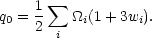(9)

From Eq. (9) we can see that, in the nearby Universe, the luminosity distances scale linearly with redshift, with H0 serving as the constant of proportionality. In the more distant Universe, DL depends to first order on the rate of acceleration/deceleration (q0) or, equivalently, on the amount and types of matter that make up the Universe. For example, since normal matter has wM = 0 and the cosmological constant has w= - 1, a universe composed of only these two forms of matter/energy has q0 =M / 2 -. In a universe composed of these two types of matter, if<M / 2, q0 is positive and the universe is decelerating. These decelerating universes have DL smaller as a function of z than their accelerating counterparts.

If distance measurements are made at a low-z and a small range of redshift at higher z (e.g., 0.3 > z > 0.5), there is a degeneracy betweenM and. It is impossible to pin down the absolute amount of either species of matter. One can only determine their relative dominance, which, at z = 0, is given by Eq. (9). However, Goobar and Perlmutter  pointed out that by observing objects over a larger range of high redshift (e.g., 0.3 > z > 1.0) this degeneracy can be broken, providing a measurement of the absolute fractions ofM and.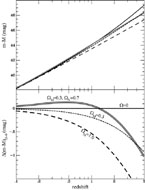Figure 1. DL expressed as distance modulus (m - M) for four relevant cosmological models;M = 0,= 0 (empty Universe, solid line);M = 0.3,= 0 (short dashed line);M = 0.3,= 0.7 (hatched line); andM = 1.0,= 0 (long dashed line). In the bottom panel the empty Universe has been subtracted from the other models to highlight the differences.

To illustrate the effect of cosmological parameters on the luminosity distance, in Fig. 1 we plot a series of models for bothand non-universes. In the top panel, the various models show the same linear behavior at z < 0.1 with models having the same H0 being indistinguishable to a few percent. By z = 0.5 the models with significantare clearly separated, with luminosity distances that are significantly further than the zero-universes. Unfortunately, two perfectly reasonable universes, given our knowledge of the local matter density of the Universe (M ~ 0.2), one with a large cosmological constant,= 0.7,M = 0.3 and one with no cosmological constant,M = 0.2, show differences of less than 25%, even to redshifts of z > 5. Interestingly, the maximum difference between the two models is at z ~ 0.8, not at large z. Fig. 2 illustrates the effect of changing the equation of state of the non-matter, dark energy component, assuming a flat universe,tot = 1. If we are to discern a dark energy component that is not a cosmological constant, measurements better than 5% are clearly required, especially since the differences in this diagram include the assumption of flatness and also fix the value ofM. In fact, to discriminate among the full range of dark energy models with time varying equations of state will require much better accuracy than even this challenging goal.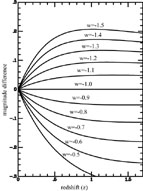Figure 2. DL for a variety of cosmological models containingM = 0.3 andx = 0.7 with a constant (not time-varying) equation of state wx. The wx = - 1 model has been subtracted off to highlight the differences between the various models